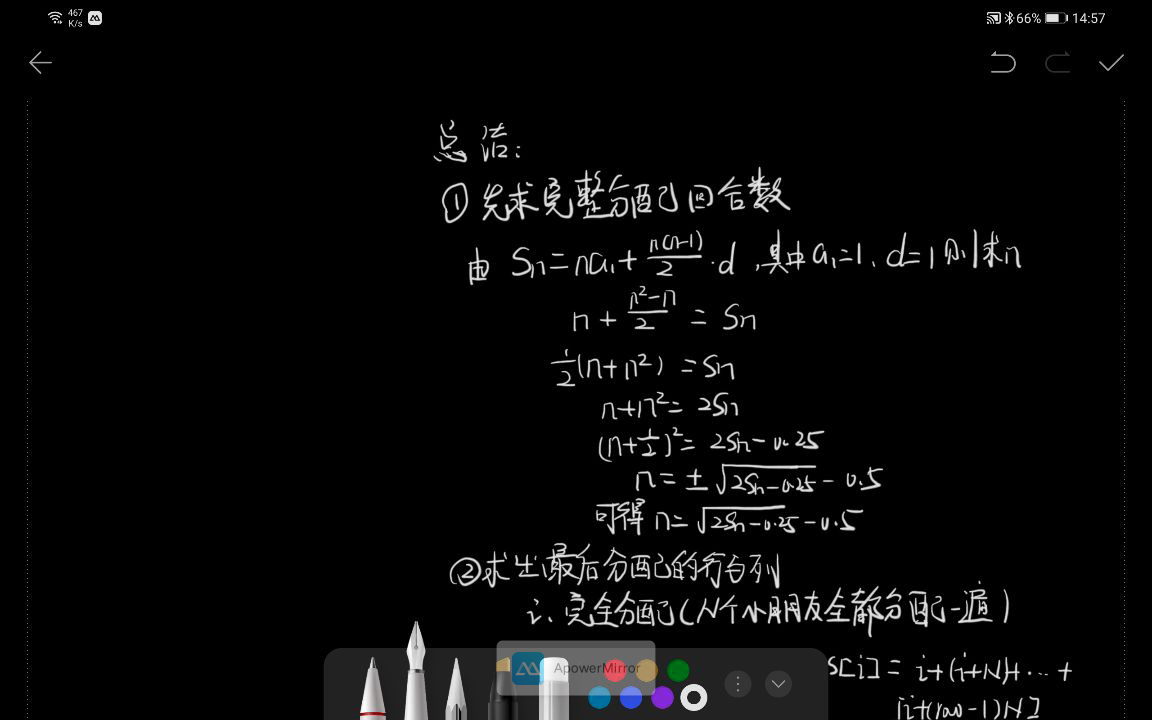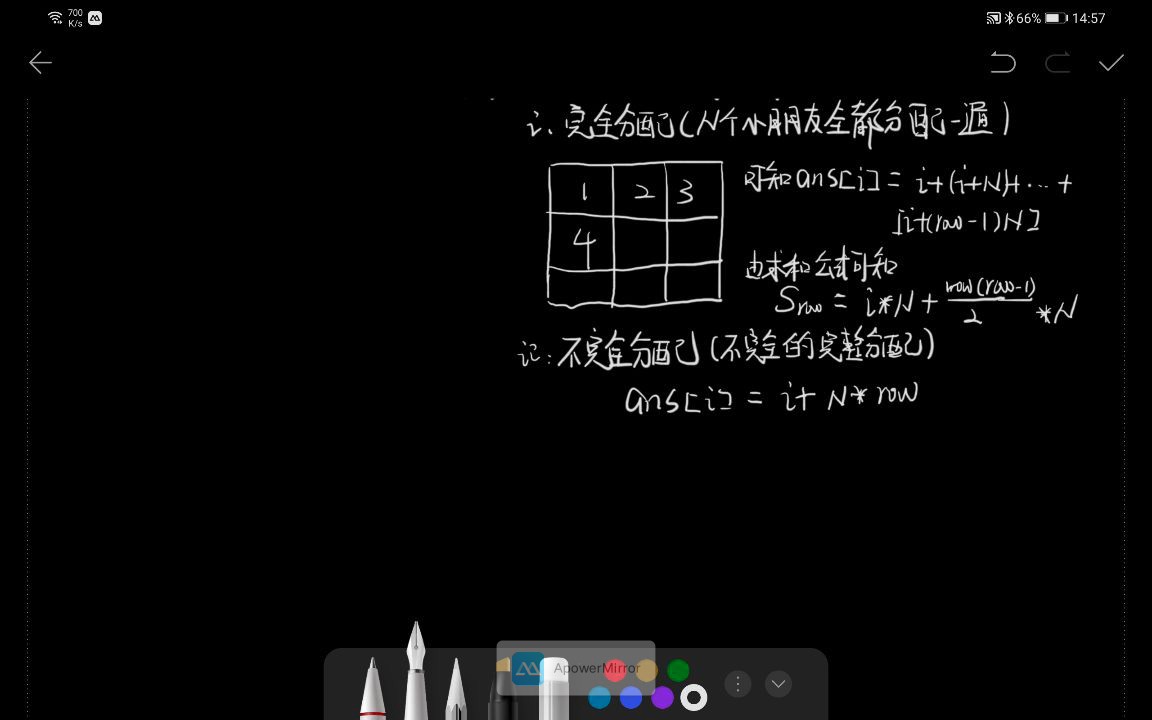## 解析

### 代码

    public int[] distributeCandies(int candies, int num_people) {
int[] ans = new int[num_people];
int pow = 0;
while (candies > 0){
for (int i = 0; i < num_people ; i++) {
if (candies >= i + 1 + num_people * pow){
candies -= i + 1 + num_people * pow;
ans[i] += i + 1 + num_people * pow;
}else {
ans[i] += candies;
candies = 0;
}

}
pow++;
}
return ans;
}

### 数学小想法(莽夫的对立面)

Sn = a1 x n+(n x n-1)/2 x d

• 求出完整分配的轮数
• 求出最后的杭和烈
• 最后加上剩余的### 代码

//等差数列求和，Sn = n*a1 + n(n-1)d /2,此处已知Sn a1 d 求 n
// n*a1 + (d * n^2 - d * n) / 2 = Sn ==> n = 土sqrt(2 * Sn + 0.25) - 0.5
public int[] distributeCandies(int candies, int num_people) {
int[] ans = new int[num_people];
//求出完整分配的项数
int n = (int) Math.floor(Math.sqrt(2*candies + 0.25) - 0.5);
//求出完整分配的数量
int sn = n + (n * (n-1)) / 2;
//剩余的糖果数
int less = candies - sn;
//推算出完整分配的行和列
//rows代表最后一行的行数
int rows = n / num_people;
// cols 代表最后一个分配的位置
int cols = n % num_people;
for (int i = 0; i < num_people ; i++) {
ans[i] = (i + 1) * rows + (int)(rows * (rows - 1) * 0.5) * num_people;
//前cols个人分配一份完整的礼物
if (i < cols) ans[i] += i + 1 + rows * num_people;
}
ans[cols] += less;
return ans;
}